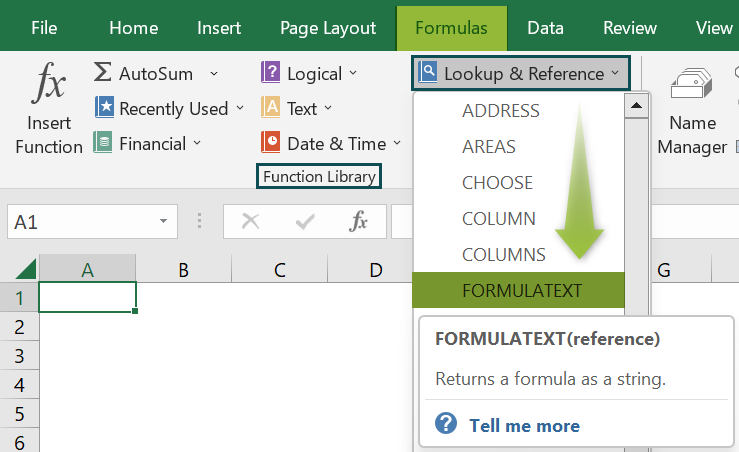# FORMULATEXT Excel FunctionArticle byExcelMojo Team## What Is FORMULATEXT Function In Excel?

The FORMULATEXT Excel function displays the formula used in a selected cell or a cell range in the form of a text. This function helps us extract the formula used in a cell instead of just viewing the result or the formula in the formula bar. The FORMULATEXT function in Excel is an inbuilt “Lookup & Reference” function, so we can insert the formula from the “Function Library” or enter it directly in the worksheet.

For example, we will apply the FORMULATEXT function to display the formula used in the image below.

Select cell C2, enter the formula =FORMULATEXT(B2), and press the “Enter” key.

The result is ‘=A2’, as shown above. In cell B2 the result is “A”, and the formula applied is ‘=A2’. However, we can only view the result and the formula in the formula bar, as shown in the second image above. So, when we apply the FORMULATEXT, the formula used in cell B2 is displayed in cell C2.

###### Key Takeaways
• The FORMULATEXT Excel function displayed the formulas used in any selected cell, just like we view in the formula bar.
• The cell reference can be from another worksheet or workbook in the argument. However, if the worksheet or workbook is not open, we will get an error.
• If the reference argument is for an entire row or column or a range or defined name containing more than one cell, then the FORMULATEXT returns the value in the upper leftmost cell of the row, column, or range.

### FORMULATEXT() Excel Formula

The syntax of the FORMULATEXT Excel formula is,

The argument of the FORMULATEXT Excel formula is,

• reference: It is a mandatory argument. It is a reference to a cell or cell range with formulas used.

### How To Use FORMULATEXT Excel Function?

We can use the FORMULATEXT Excel function in 2 ways, namely,

1. Access from the Excel ribbon.
2. Enter in the worksheet manually.

#### Method #1 – Access from the Excel ribbon

Choose an empty cell for the output → select the “Formulas” tab → go to the “Function Library” group → click the “Lookup & Reference” option drop-down → select the “FORMULATEXT” function, as shown below.

The “Function Arguments” window appears. Enter the argument in the “Reference” field → click “OK”, as shown below.

#### Method #2 – Enter in the worksheet manually

1. Select an empty cell for the output.
2. Type =FORMULATEXT( in the selected cell. [Alternatively, type =F or =FO and double-click the FORMULATEXT function from the list of suggestions shown by Excel.]
3. Enter the arguments as only cell references and close the brackets.
4. Press the “Enter” key.

Let us take a basic example to understand its application.

We will find the output formula using FORMULATEXT Excel function for the date.

In the table, the data is,

• Column A contains the Value.
• Column B displays the Output.

The steps to find the value using FORMULATEXT formula are,

1. Select cell B2, and enter the formula =FORMULATEXT(A2), i.e., the reference cell value.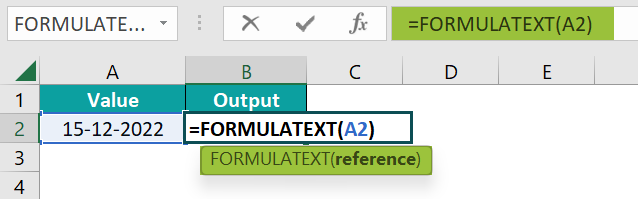2. Press the “Enter” key. The result is “=TODAY()”, as shown below.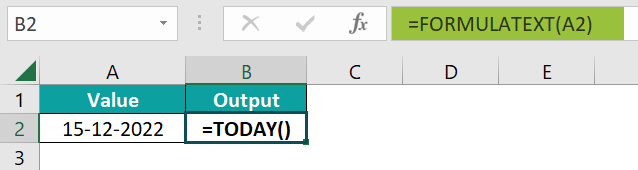### Examples

We will understand some advanced scenarios using the FORMULATEXT Excel function examples.

#### Example #1

The succeeding example depicts the sales and cost price of some items, and it calculates the profit earned. We will fetch the output’s formula using the FORMULATEXT function.

In the table, the data is,

• Column A contains the Cost Price.
• Column B contains the Selling Price.
• Here, column C contains the Profit.
• Column D contains the Output.

The steps to find the value using FORMULATEXT formula are,

• Step 1: Select cell D2, enter the formula =FORMULATEXT(C2), and press the “Enter” key. The result is “=A2-B2”, as shown below.
• Step 2: Drag the formula from cell D2 to D5 using the fill handle in Excel. The output is shown below.

#### Example #2

The succeeding example depicts the values and calculated average. We will fetch the output’s formula using the FORMULATEXT function.

In the table, the data is,

• Column A contains the Value.
• Here, column B contains the Average.
• Column C contains the Output.

The procedure to find the formula using the FORMULATEXT formula is,

Select cell C2, enter the formula =FORMULATEXT(B2), and press the “Enter” key.

The result is “=AVERAGE(A2:A5)”, as shown above.

#### Example #3

The succeeding example depicts the value and calculated sum. We will fetch the output’s formula using the FORMULATEXT function.

In the table, the data is,

• Column A contains the Value.
• Here, column B contains the Sum.
• Column C contains the Output.

The procedure to find the formula using the FORMULATEXT formula is,

Select cell C2, enter the formula =FORMULATEXT(B2), and press the “Enter” key.

The result is “SUM(A2:A3)”, as shown above.

### Important Things To Note

• The “#N/A” error occurs,
• If the cell reference is not a result of a formula.
• When the characters in the formula in the cell should not exceed 8192 characters.
• If the worksheet can’t display the formula.
• The “#VALUE!” error occurs when the input data types are invalid.

1. What does the FORMULATEXT Excel function mean?

The FORMULATEXT function converts and returns the output of a given formula in the text string format.

The FORMULATEXT function is =FORMULATEXT(reference)

For example, we will fetch the output formula using the FORMULATEXT function.

In the table, the data is,
Column A contains the Value.
Here, column B contains the Formula Cells.
Column C contains the Output.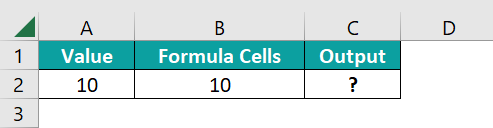The procedure to find the formula using the FORMULATEXT formula is,
Select cell C2, enter the formula =FORMULATEXT(B2), and press the “Enter” key.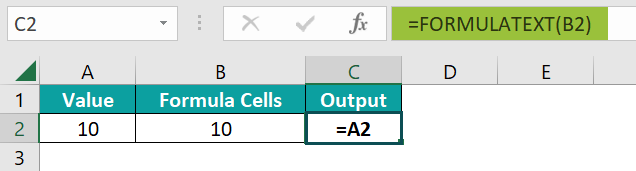The result is “=A2”, as shown above.

2. How to insert the FORMULATEXT Excel function?

We can insert the FORMULATEXT function as follows:
1. Select an empty cell for the output.
2. Type =FORMULATEXT( in the selected cell. [Alternatively, type =F and double-click the FORMULATEXT function from the list of suggestions shown by Excel.]
3. Enter the arguments as only cell references and close the brackets.
4. Press the “Enter” key.

For example, the image depicts the value, and we will fetch the output formula using the FORMULATEXT function.

In the table, the data is,
Column A contains the Value.
Here, column B contains the Formula Cell.
Column C contains the Output.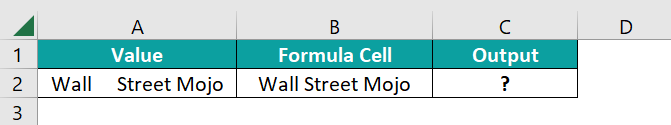The procedure to find the formula using the FORMULATEXT formula is,
Select cell C2, enter the formula =FORMULATEXT(B2), and press the “Enter” key.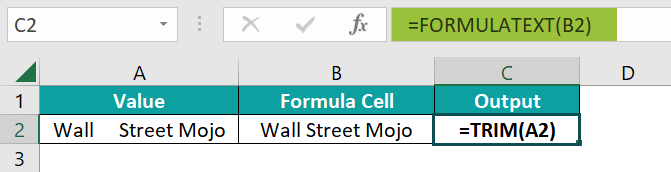The result is “TRIM(A2)”, as shown above.

3. Where is the FORMULATEXT Excel function?

The FORMULATEXT function is in the “Formulas” tab, as shown below.

Choose an empty cell for the output → select the “Formulas” tab → go to the “Function Library” group → click the “Lookup & Reference” option drop-down → select the “FORMULATEXT” function, as shown below.